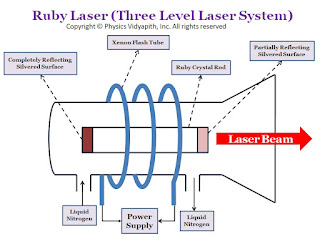Showing posts with label Capacitors. Show all posts
Showing posts with label Capacitors. Show all posts

## Energy Stored in a Charged Capacitor

Description→

When a capacitor is charged, the work is done by charged battery (i.e the chemical energy of the battery is used to charge the capacitor). As the capacitor gets charged, the potential difference between its plates increases. Due to this increase in the potential difference between the plates, the battery has to give the same amount of charge to the capacitor. Because of that, the battery has to do more and more work.

The total amount of work done in charging the capacitor is stored in the form of electric potential energy in between the capacitor plates. This energy is retrieved as heat when the capacitor is discharged through a resistance.

Derivation→

Let us consider, a capacitor of capacitance $C$with a potential difference of $V$ between the plates.

In the process of charging, electrons are transferred from the positive to negative, unit each plate acquires an amount of charge $q$. Suppose during the process of charging, we increase the charge from $q'$ to $q'+dq'$ by transferring an amount of negative charge $dq'$ from the positive to the negative plate. the work done

$dW=V' \: dq'$

$dW=\frac{q'}{C} \: dq'$

Therefore, the total work done in charging the capacitor from the uncharged state (i.e. zero charge in the capacitor) to the final charge $q$ will be

$W=\int dW$

$W=\int_{0}^{q} \frac{q'}{C} \: dq'$

$W=\frac{1}{2}\left[ \frac{q'^{2}}{C} \right]^{q}_{0}$

$W=\frac{1}{2}\left[ \frac{q^{2}}{C} \right]$

$W=\frac{1}{2}\:C \: V^{2}$

This work done is stored in the form of potential energy $U$ within the capacitor. Thus

$U=\frac{1}{2}\:C \: V^{2}$

 $U=\frac{1}{2}\frac{q^{2}}{C}$

Energy Stored in Combination of Capacitor→

If many capacitors are combined in series, or in parallel, the total potential energy stored in either combination is equal to the sum of the potential energies stored in the individual capacitor. This follows from the combination of the capacitor's expressions:

The potential energy is stored in a series combination→

The potential energy stored in a series combination (here $q$ is constant) is

$U=\frac{1}{2}\frac{q^{2}}{C}$

For series combination the capacitance of capacitor i.e. $\frac{1}{C}=\frac{1}{C_{1}}+\frac{1}{C_{2}}+\frac{1}{C_{2}}+..........$. Now substitute the value of $\frac{1}{C}$ in above equation

$U=\frac{1}{2}q^{2} \frac{1}{C_{1}}+\frac{1}{C_{2}}+\frac{1}{C_{2}}+..........$

 $U= \left( \frac{1}{2}q^{2} \frac{1}{C_{1}}+\frac{1}{2}q^{2} \frac{1}{C_{2}}+ \frac{1}{2}q^{2} \frac{1}{C_{2}}+.......... \right)$

 $U=U_{1}+U_{2}+U_{3}+.........$

The potential energy is stored in a parallel combination→

The potential energy is stored in a parallel combination (here $V$ is constant) is

$U=\frac{1}{2}CV^{2}$

For parallel combination the capacitance of capacitor i.e. $C=C_{1}+C_{2}+C_{3}+......$. Now substitute the value of $C$ in the above equation

$U=\frac{1}{2} \left( C_{1}+C_{2}+C_{3}+........... \right) V^{2}$

 $U= \frac{1}{2} C_{1} V^{2} + \frac{1}{2} C_{2} V^{2} +\frac{1}{2} C_{3}V^{2} +.....$

 $U=U_{1}+U_{2}+U_{3}+......$

## Force between the plates of a Charged Parallel Plate Capacitor

Derivation and Description→

Consider, A parallel plate capacitor with a charge $+q$ on one of its plates and $-q$ on the other plate. Let initially the plates of the capacitor are almost, but not quite touching. Due to opposite polarity, there is an attractive force $F$ between the plates. Now If these plates are gradually pulling and apart to a distance $d$, in such a way that $d$ is still small compared to the linear dimension of the plates, then the approximation of a uniform field between the plates is maintained and thus the force remains constant.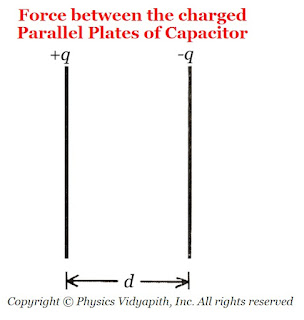The force between the charged parallel plates of the capacitor

Now the work done in separating the plates from near $0$ to $d$,

$W=F.d \qquad(1)$

This work done $(W)$ is stored as electrostatic potential energy $(U)$ between the plates, i.e.

$U=\frac{1}{2}q V$

But $V=E.d$, then the above equation can be written as

$U=\frac{1}{2}q \: E \: d \qquad(2)$

But the equation $(1)$ and equation $(2)$ both are equal then

$F.d=\frac{1}{2}q \: E \: d$

 $F=\frac{1}{2}q \: E$

The factor $\frac{1}{2}$ arises because just outside the conducting plates the field is $E$ and inside the plates, the field is zero. So the average value $\frac{E}{2}$ contributes to the force.

## Capacitance of a Parallel Plate Capacitor Partly Filled with Dielectric Slab between Plates

Derivation→ Let us consider,

The charge on a parallel-plate capacitor = $q$
The area of parallel-plate = $A$
The distance between the parallel-plate = $d$
The dielectric constant of the slab of a material =$K$
The thickness of the material =$t$
The vacuum (or air) between the plates =$(d-t)$ The surface charge density on the plates= $\sigma$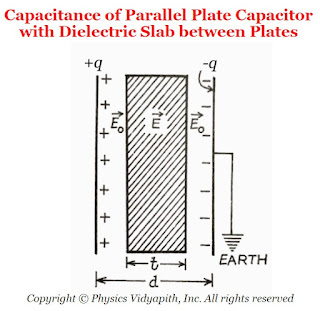The capacitance of Parallel Plate Capacitor with Dielectric Slab between Plates
The electric field in the air between the plates is

$E_{\circ}=\frac{\sigma}{\epsilon_{\circ}}$

$E_{\circ}=\frac{q}{\epsilon_{\circ}\: A} \qquad(1)$

The electric field in the dielectric material

$E=\frac{q}{\epsilon_{\circ}\: K\: A} \qquad(2)$

The potential difference between the plates

$V=E_{\circ}\left( d-t \right)+E\:t \qquad(3)$

Now substitute the value of $E_{\circ}$ and $E$ in the equation $(3)$, Then we get

$V=\frac{q}{\epsilon_{\circ}\: A} \left( d-t \right)+ \frac{q}{\epsilon_{\circ}\: K\: A} \:t$

$V=\frac{q}{\epsilon_{\circ}\: A} \left[ \left( d-t \right)+ \frac{t}{K} \right] \qquad$

The capacitance of the capacitor is

$C=\frac{q}{V}$

Now substitute the value of electric potential $V$ in the above equation then

$C= \frac{q}{\frac{q}{\epsilon_{\circ}\: A} \left[ \left( d-t \right)+ \frac{t}{K} \right]}$

 $C= \frac{\epsilon_{\circ}\: A}{\left[ \left( d-t \right)+ \frac{t}{K} \right]}$

Since $K>1$, the 'effective' distance between the plates becomes less than $d$ and so the capacitance increases.

Special Cases→

1. When the dielectric slab is completely filled between the parallel plates i.e. $t=d$ then the capacitance between the parallel plates

 $C= \frac{K \: \epsilon_{\circ}\: A}{d}$

2. When there is a vacuum (or air) between the parallel plates i.e. $t=0$, then the capacitance between the parallel plates

 $C_{\circ}= \frac{K \: \epsilon_{\circ}\: A}{d}$

3. When there is a slab of metal whose dielectric constant is infinity (K=∞). If the thickness of the slab is t between the parallel plates, then the capacitance between the parallel plates

 $C= \frac{K \: \epsilon_{\circ}\: A}{d-t}$

4. When the slabs of dielectric constants $K_{1},K_{2},K_{3},K_{4}...........$ and respective thickness $t_{1},t_{2},t_{3},t_{4},........$ is placed in the entire space between the parallel-plates, then the capacitance between the plates

 $C=\frac{\epsilon_{\circ} A}{d- \left( t_{1}+t_{2}+t_{3}+.... \right)+ \left( \frac{t_{1}}{K_{1}}+\frac{t_{2}}{K_{2}}+\frac{t_{3}}{K_{3}}+....... \right)}$

But $d=t_{1}+t_{2}+t_{3}+....$

 $C=\frac{\epsilon_{\circ} A}{ \left( \frac{t_{1}}{K_{1}}+\frac{t_{2}}{K_{2}}+\frac{t_{3}}{K_{3}}+..... \right)}$

## Parallel Plate Air Capacitor and Its Capacitance

Parallel Plate Air Capacitor→

A parallel-plate capacitor consists of two long, plane, metallic plates mounted on two insulating stands and placed at a small distance apart in a vacuum (or air). The plates are exactly parallel to each other.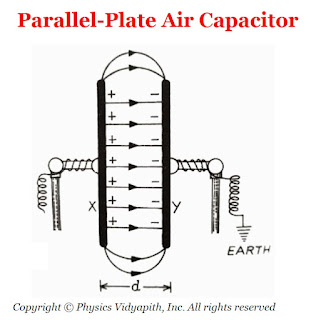Parallel Plate Air Capacitor
Derivation of the capacitance of parallel plate capacitor in the air→

Let us consider, Two plates $X$ and $Y$ are separated at a small distance $d$ in the vacuum (or air). If the area of plates is $A$ and the plates $X$ and $Y$ have charge $+q$ and $-q$ respectively. If the surface charge density on each plate is $\sigma$ then electric field intensity at a point between two parallel plates is

$E=\frac{\sigma}{\epsilon_{\circ}}$

$E=\frac{q}{\epsilon_{\circ}A}\qquad(1) \qquad (\because \sigma=\frac{q}{A})$

The potential difference between the parallel plates capacitor in air (or vacuum) is

$V=E.d$

Now substitute the value of $E$ from equation$(1)$ in the above equation

$V=\frac{q}{\epsilon_{\circ}A}.d \qquad(2)$

The capacitance of the parallel plate capacitor in air (or vacuum) is

$C_{\circ}=\frac{q}{V} \qquad(3)$

From equation $(2)$ and equation $(3)$

$C_{\circ}=\frac{q}{\frac{q}{\epsilon_{\circ}A}.d}$

 $C_{\circ}=\frac{\epsilon_{\circ}A}{d}$

If the space between the plates is filled with some dielectric medium of dielectric constant $K$, then the electric field between the plates is

$E=\frac{\sigma}{K \: \epsilon_{\circ}}$

The Potential difference between the parallel plates when dielectric medium $(K)$ is present between them

$V=\frac{q}{K \: \epsilon_{\circ} \: A}.d$

Now, The capacitance of the parallel-plate capacitor when the dielectric medium $(K)$ is present between them

 $C=\frac{K\: \epsilon_{\circ} \: A}{d}$

## Potential Energy of a Charged Conductor

Derivation →

Let us consider a conductor of capacitor $C$ is given a charge $+q$ then the electric potential

$V=\frac{q}{C} \qquad(1)$

The work done in charging the conductor is stored as potential energy in the electric field in the vicinity of the conductor.

Let bring a small charge $dq$ from infinity to the surface of the conductor. Then the work done will be

$dW=V\:dq \qquad(2)$

From equation $(1)$ and equation $(2)$

$dW=\frac{q}{C} \:dq$

Therefore, the work done in increasing the charge on the conductor from $0$ to $q$ is

$W=\int dW$

$W=\frac{1}{C} \int_{0}^{q} q dq$

$W=\frac{1}{C} \left[ \frac{q^{2}}{2} \right]^{q}_{0}$

$W=\frac{1}{2} \frac{q^{2}}{C}$

This work is stored in the form of electric potential energy $U$. Then

 $U=\frac{1}{2} \frac{q^{2}}{C}$

 $U=\frac{1}{2} C V^{2}$

 $U=\frac{1}{2} q V$

## Capacitance of an Isolated Spherical Conductor

Derivation →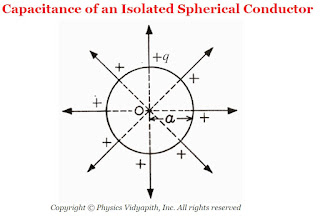Capacitance of an Isolated Spherical Conductor

Let us consider an isolated spherical conductor of radius $a$ is placed in a vacuum or air. Let a charge $+q$ be given to the sphere and this charge is distributed uniformly on the surface of conducting sphere. Then the electric potential at the surface of the conducting sphere is

$V=\frac{1}{4\pi \epsilon_{\circ}} \frac{q}{a} \qquad(1)$

So the capacitance of the sphere

$C=\frac{q}{V} \qquad(2)$

Now substitute the value of $V$ in equation $(2)$ Then we get

$C=\frac{q}{\frac{q}{4\pi \epsilon_{\circ}a}}$

 $C=4\pi \epsilon_{\circ}a$

Thus, The capacitance of a spherical conductor is directly proportional to its radius. i.e If the radius of conducting sphere is large then the sphere will hold a large amount of the given charge without running up too high a voltage.

Unit: The unit of capacitance is "farad" i.e. $F$

### Principle, Construction and Working of the Ruby Laser